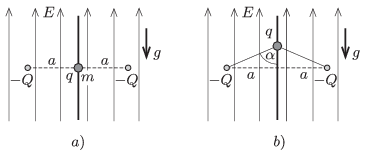Mathematical and Physical Journal
for High Schools
Issued by the MATFUND Foundation
 Already signed up? New to KöMaL?

#Problem P. 4798. (January 2016)

P. 4798. In a region of uniform, vertically upward electric field the magnitude of the electric field strength is $\displaystyle E$. In this field there are two fixed charges of charge $\displaystyle -Q$ at a distance of $\displaystyle 2a$ along a horizontal line. At the midpoint of the line segment between the two charges there is a small bead on a vertical thread (as shown in figure $\displaystyle a)$). The charge on the bead is $\displaystyle q>0$ and its mass is $\displaystyle m$. The bead is released from rest. (It can move without friction along the thread.) When the bead reaches the furthest position from its starting point, the two charges and the bead will be at the vertices of an isosceles triangle of vertex angle $\displaystyle 2\alpha=150^\circ$, as shown in figure $\displaystyle b)$.$\displaystyle a)$ Find $\displaystyle q$.

$\displaystyle b)$ What will the greatest speed of the bead be?

$\displaystyle c)$ What should the least initial speed given to the bead be, in order that it can reach any (distant) position?

Data: $\displaystyle E=10^4$ N/C, $\displaystyle a=10$ cm, $\displaystyle Q=10^{-8}$ C, $\displaystyle \alpha=75^\circ$, $\displaystyle m=0.1$ g.

(5 pont)

Deadline expired on February 10, 2016.

### Statistics:

 30 students sent a solution. 5 points: Asztalos Bogdán, Balogh Menyhért, Bekes Nándor, Blum Balázs, Büki Máté, Csorba Benjámin, Fajszi Bulcsú, Forrai Botond, Iván Balázs, Kasza Bence, Kovács Péter Tamás, Körmöczi Dávid, Németh Flóra Boróka, Páhoki Tamás, Sal Kristóf, Szentivánszki Soma , Tomcsányi Gergely, Tóth Adrián. 4 points: Fekete Balázs Attila, Kormányos Hanna Rebeka, Németh 777 Róbert, Pázmán Előd. 3 points: 4 students. 2 points: 2 students. 1 point: 1 student. 0 point: 1 student.

Problems in Physics of KöMaL, January 2016